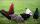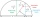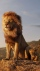# Functions + expression of a variable from the formula - math problems

#### Number of problems found: 271

• Trapezoid - central medianThe central median divides the trapezoid into two smaller trapezoids. Determines the ratio of their contents.
• DecibelBy what percentage does the sound intensity increase if the sound intensity level increases by 1 dB?
• Cube edgesIf the edge length of the cube increases by 50%, how does the volume of this cube increase?
• Sphere cutA sphere segment is cut off from a sphere k with radius r = 1. The volume of the sphere inscribed in this segment is equal to 1/6 of the volume of the segment. What is the distance of the cutting plane from the center of the sphere?
• Triangle perimeterCalculate the triangle perimeter whose sides are in ratio 3: 5: 7 and the longest side is 17.5 cm long.
• Integer sidesA right triangle with an integer length of two sides has one leg √11 long. How much is its longest side?
• Sales offThe price has decreased by 20%. How many percents do I have to raise the new price to be the same as before the cut?
• The priceThe price of the land increased by 17%. What was the original price of the land if it now costs 46800 €?
• One and a half chickenOne and a half chicken bears one and a half egg in one and a half day. How many eggs can withstand from 6 chicken in 6 days?
• Interior anglesCalculate the interior angles of a triangle that are in the ratio 2: 3: 4.
• Find theFind the number x, which if it increases by 2, then its square increases by 21 percent.
• Volume of sphereHow many times does the volume of a sphere increase if its radius increases 2 ×?
• The sum 6The sum of the 17 different natural numbers is 153. Determine the sum of the two largest ones.
• CombinationsIf the number of elements increase by 3, it increases the number of combinations of the second class of these elements 5 times. How many are the elements?
• Cube into sphereThe cube has brushed a sphere as large as possible. Determine how much percent was the waste.
• Harmonic meanIf x, y, z form a harmonic progression, then y is the harmonic mean of x and z. Find the harmonic mean of the numbers 6 and 5.
• LionsIf 8 lions is 40% how many would 75% be?How many times does the surface of a sphere decrease if we reduce its radius twice?
• Unknown number 24f we add 20, we get 50% of its triple. What is this unknown number?
• The aspect ratioThe aspect ratio of the rectangular triangle is 13: 12: 5. Calculate the internal angles of the triangle.

Do you have an interesting mathematical word problem that you can't solve it? Submit a math problem, and we can try to solve it.

We will send a solution to your e-mail address. Solved examples are also published here. Please enter the e-mail correctly and check whether you don't have a full mailbox.

Please do not submit problems from current active competitions such as Mathematical Olympiad, correspondence seminars etc...

Functions - math word problems. Expression of a variable from the formula - math word problems.